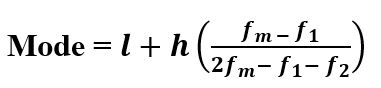Open in App
Not now

# scipy stats.mode() function | Python

• Last Updated : 11 Feb, 2019

`scipy.stats.mode(array, axis=0)` function calculates the mode of the array elements along the specified axis of the array (list in python).

Its formula –```where,
l : Lower Boundary of modal class
h : Size of modal class
fm : Frequency corresponding to modal class
f1 : Frequency preceding  to modal class
f2 : Frequency proceeding to modal class```

Parameters :
array : Input array or object having the elements to calculate the mode.
axis : Axis along which the mode is to be computed. By default axis = 0

Returns : Modal values of the array elements based on the set parameters.

Code #1:

 `# Arithmetic mode  ``from` `scipy ``import` `stats``import` `numpy as np `` ` `arr1 ``=` `np.array([[``1``, ``3``, ``27``, ``13``, ``21``, ``9``],``                ``[``8``, ``12``, ``8``, ``4``, ``7``, ``10``]]) ``  ` ` ` `print``(``"Arithmetic mode is : \n"``, stats.mode(arr1)) `

Output :

```Arithmetic mode is :
ModeResult(mode=array([[1, 3, 8, 4, 7, 9]]), count=array([[1, 1, 1, 1, 1, 1]]))```

Code #2: With multi-dimensional data

 `# Arithmetic mode ``from` `scipy ``import` `stats``import` `numpy as np `` ` `arr1 ``=` `[[``1``, ``3``, ``27``], ``        ``[``3``, ``4``, ``6``], ``        ``[``7``, ``6``, ``3``], ``        ``[``3``, ``6``, ``8``]] ``   ` `print``(``"Arithmetic mode is : \n"``, stats.mode(arr1)) `` ` `print``(``"\nArithmetic mode is : \n"``, stats.mode(arr1, axis ``=` `None``)) `` ` `print``(``"\nArithmetic mode is : \n"``, stats.mode(arr1, axis ``=` `0``)) `` ` `print``(``"\nArithmetic mode is : \n"``, stats.mode(arr1, axis ``=` `1``)) `

Output :

```Arithmetic mode is :
ModeResult(mode=array([[3, 6, 3]]), count=array([[2, 2, 1]]))

Arithmetic mode is :
ModeResult(mode=array(), count=array())

Arithmetic mode is :
ModeResult(mode=array([[3, 6, 3]]), count=array([[2, 2, 1]]))

Arithmetic mode is :
ModeResult(mode=array([,
,
,
]), count=array([,
,
,
]))```

My Personal Notes arrow_drop_up